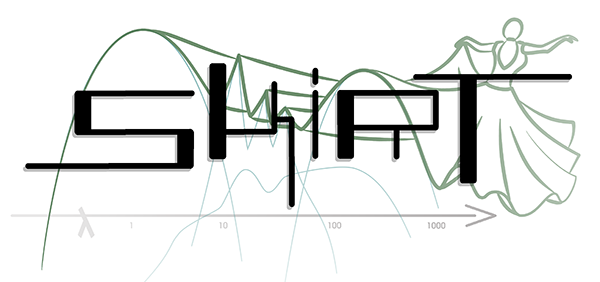SKIRT 9 advanced radiative transfer in dusty systems
Spatial grids

# Spatial model characteristics

In a SKIRT simulation, the spatial distributions of the model are defined as part of the SourceSystem (for the radiation sources) and the MediumSystem (for the transfer medium). The distributions may be specified through one or more of the built-in geometries (Geometry) or it may be imported from a snapshot generated by a hydrodynamical simulation.

In all cases, the spatial characteristics of the model are internally described in a three-dimensional, right-handed Cartesian coordinate system, with some provisions for keeping track of specific spherical or cylindrical symmetries where appropriate. In many cases the model domain is centered on the origin of the coordinate system, but this is not a requirement. In fact, facilities such as the OffsetGeometryDecorator class allow easily defining off-center structures.

A model is considered to be spherically or cylindrically symmetric if all sources and media components show the corresponding symmetry. By definition, a spherically symmetric model is centered on the origin, and a cylindrically symmetric model is centered on the vertical coordinate axis.

Incidentally, SKIRT internally always uses SI units (i.e. meter for distances and lengths), regardless of the units selected by the user for input/output purposes.

# Spatial grids

A SKIRT simulation employs an instance of the SpatialGrid class to spatially discretize the transfer medium of the model. Properties such as the material density and the calculated radiation field are considered to be constant within each cell of the grid. It is thus necessary to provide a sufficiently fine grid in the relevant areas of the model. On the other hand, simulation runtime and memory requirements scale with the number of cells, so it is often necessary to minimize the number of cells in less relevant areas of the model.

SKIRT offers a range of spatial grid options to help accomplish this balance. The following (incomplete) diagram illustrates the SpatialGrid class hierarchy (connections starting with a triangle denote inheritance).The SphereSpatialGrid subclasses are defined in spherical coordinates and their configuration space is a sphere centered on the origin. Likewise, the CylinderSpatialGrid subclasses are defined in cylindrical coordinates and their configuration space is a cylinder centered on the vertical coordinate axes. The BoxSpatialGrid subclasses are defined in Cartesian coordinate and their configuration space is a cuboid lined up with the coordinate axes (but not necessarily centered on the origin).

The Sphere1DSpatialGrid class provides spatial grids in which the grid cells are concentric shells. It can be used only when the model is spherically symmetric.

The Sphere2DSpatialGrid class provides axisymmetric spatial grids based on spherical coordinates. The grid is defined in the meridional $$(r,\theta)$$ plane and rotated around the vertical coordinate axis. The Cylinder2DSpatialGrid class provides axisymmetric spatial grids based on cylindrical coordinates. The grid is defined in the meridional $$(R,Z)$$ plane and rotated around the vertical coordinate axis. These grids can be used only when the model is cylindrically (or spherically) symmetric.

The CartesianSpatialGrid class provides three-dimensional spatial grids based on a regular cartesian grid. Each cell is a cuboid lined up with the coordinate axes, although cells do not necessarily all have the same size or form factor. The PolicyTreeSpatialGrid class offers a spatial grid in which the cubdoidal domain is recursively divided into two or eight subcells until the resulting cells meet some predefined local criteria (e.g. contain less than a given fraction of the total material mass). This allows to place many small cells in relevant regions and fewer larger cells in other regions. The VoronoiSpatialGrid class uses a Voronoi tesselation to discretize the spatial domain; each cell is a convex polyhedron. The BoxSpatialGrid subclasses can be used regardless of any symmetries in the model.

The four classes indicated in the diagram with a blue border implement spatial grids that need an instance of a Mesh subclass to define the position of the cell borders in each dimension, as described in the next section.

# Meshes

In SKIRT, an instance of a Mesh subclass characterizes a one-dimensional mesh over the unit interval $$[0,1]$$. A mesh is essentially a partition of this interval into $$N$$ bins defined by an ordered sequence of $$N+1$$ mesh points $${ti}$$, with $$t_0=0$$ and $$t_\mathrm{N}=1$$. The different Mesh subclasses illustrated in the following (incomplete) diagram define various mesh point distributions (connections starting with a triangle denote inheritance).The LinMesh class represents meshes with a linear distribution of the mesh points. The PowMesh class represents meshes with with a power-law distribution of the mesh points. The bin sizes form a geometric series, i.e. each bin is a constant factor larger than the previous one. The distribution is characterized by a single parameter, the bin width ratio between the last and the first bin. The SymPowMesh class represents meshes with a symmetric power-law distribution of the mesh points. The bin sizes form a geometric series starting from the innermost bin and moving outwards symmetrically. If the number of bins is odd, there is one innermost bin; if it is even, there are two equal-size innermost bins. The LogMesh class represents meshes with a logarithmic distribution of the mesh points. The first bin covers the interval $$[0,t_\mathrm{c}]$$ and the widths of the remaining bins are distributed logarithmically over the interval $$[t_\mathrm{c},1]$$, where $$t_\mathrm{c}$$ is a user-configurable parameter.

The object holding (and using) a Mesh instance is responsible for applying a linear transform from the unit interval $$[0,1]$$ on which the mesh is defined to the desired interval. A MoveableMesh may be scaled and shifted to an arbitrary interval $$[x_\mathrm{min},x_\mathrm{max}]$$. An AnchoredMesh should not be shifted, i.e. it can only be scaled to a interval $$[0,x_\mathrm{max}]$$ anchored at the origin. This is appropriate, for example, for logarithmic distributions where the first bin has special meaning.

The radial dimensions of a spatial grid accept any type of Mesh, including AnchoredMesh and MoveableMesh subclasses, while the cartesian dimensions of a spatial grid require a MoveableMesh subclass (because an offset may need to be applied to the grid, in addition to a scale).